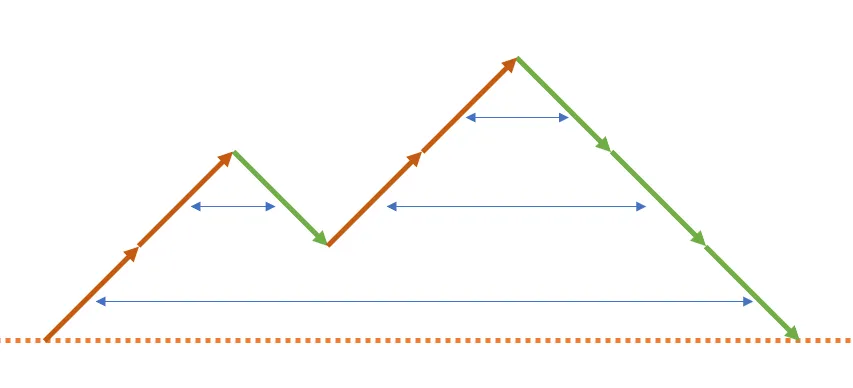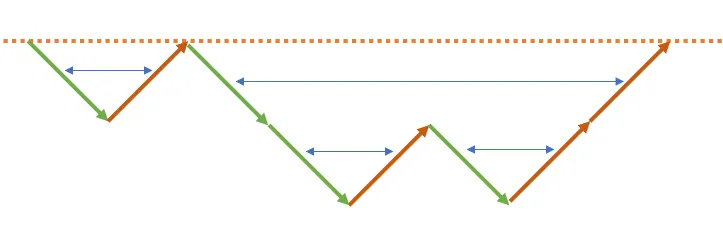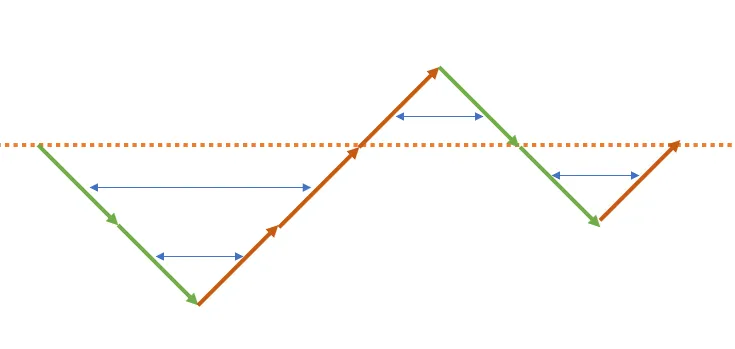Educational Codeforces Round 149 (Rated for Div. 2)

D. Bracket Coloring

https://codeforces.com/contest/1837/problem/D

2 seconds / 512 megabytes

standard input / standard output

## Problem

A regular bracket sequence is a bracket sequence that can be transformed into a correct arithmetic expression by inserting characters "1" and "+" between the original characters of the sequence. For example:

• the bracket sequences "()()" and "(())" are regular (the resulting expressions are: "(1)+(1)" and "((1+1)+1)");
• the bracket sequences ")(", "(" and ")" are not.
A bracket sequence is called beautiful if one of the following conditions is satisfied:
• it is a regular bracket sequence;
• if the order of the characters in this sequence is reversed, it becomes a regular bracket sequence.

For example, the bracket sequences "()()", "(())", ")))(((", "))()((" are beautiful.

You are given a bracket sequence $s$. You have to color it in such a way that:

• every bracket is colored into one color;
• for every color, there is at least one bracket colored into that color;
• for every color, if you write down the sequence of brackets having that color in the order they appear, you will get a beautiful bracket sequence.

Color the given bracket sequence $s$ into the minimum number of colors according to these constraints, or report that it is impossible.

## Input

The first line contains one integer $t$ ($1 \le t \le 10^4$) — the number of test cases.

Each test case consists of two lines. The first line contains one integer $n$ ($2 \le n \le 2 \cdot 10^5$) — the number of characters in $s$. The second line contains $s$ — a string of $n$ characters, where each character is either "(" or ")".

Additional constraint on the input: the sum of $n$ over all test cases does not exceed $2 \cdot 10^5$.

## Output

For each test case, print the answer as follows:

• if it is impossible to color the brackets according to the problem statement, print $-1$;
• otherwise, print two lines. In the first line, print one integer $k$ ($1 \le k \le n$) — the minimum number of colors. In the second line, print $n$ integers $c_1, c_2, \dots, c_n$ ($1 \le c_i \le k$), where $c_i$ is the color of the $i$-th bracket. If there are multiple answers, print any of them.

Input

4
8
((())))(
4
(())
4
))((
3
(()

Output

2 2 2 1 2 2 2 1
1
1 1 1 1
1
1 1 1 1
-1

## 题解

#### 重点二：颜色数量## 代码

#include <bits/stdc++.h>
#define endl '\n'

using namespace std;

void solve()
{
int n;
cin >> n;
string s;
cin >> s;
vector<int> a(n + 1);
bool pos = false, neg = false;
for (int i = 0; i < n; i++)
{
if (s[i] == '(')
a[i + 1] = a[i] + 1;
else
a[i + 1] = a[i] - 1;
if (a[i] > 0)
pos = true;
if (a[i] < 0)
neg = true;
}
if (a.back() != 0)
{
cout << -1 << endl;
return;
}
if (!(pos && neg))
{
cout << 1 << endl;
for (int i = 0; i < n; i++)
cout << 1 << ' ';
cout << endl;
}
else
{
cout << 2 << endl;
for (int i = 0; i < n; i++)
{
if (a[i] >= 0 && a[i + 1] >= 0)
cout << 1 << ' ';
else
cout << 2 << ' ';
}
cout << endl;
}
}

signed main()
{
ios::sync_with_stdio(false);
cin.tie(0);
cout.tie(0);
int t;
cin >> t;
while (t--)
solve();
return 0;
}